# Using XLSTAT functions within an Excel sheet

2017-10-06

## Available functions in XLSTAT

XLSTAT allows you to directly use some XLSTAT functions within your Excel sheet.

The functions that are currently available solve numerical issues that have been found with the built-in Excel functions, or complement the set of Excel statistical functions. They are accessible from the Insert function menu of MS Excel.

Watch this video to see how you can access the XLSTAT functions.

The following functions are available:

Function Description Parameters
XLSTAT_Linest(Y, X,WgIntercept0,Tolerance) Computes a series of outputs of the linear regression of Y with Xs as explanatory variables. To access the elements of the output table, use the Index function of Excel. XLSTAT_Linest uses the same output format as the Excel Linest function (see the Linest function description in the Excel help). The advantage of XLSTAT_Linest in comparison with Linest is that you can use it with up to 2000 variables, and define observation weights, and filter variables using the tolerance criterion. Y: a column that contains the data corresponds to the dependent variable. Missing values are not accepted. X: a series of columns, where each column corresponds to an explanatory variable. Wg: optional input; a column that contains the weights of the observations. intercept0: optional input; a boolean (TRUE or FALSE) that tells if you want to fix the intercept to 0 or not. Tolerance: optional input; a value between 0 and 1 to filter out explanatory variables that are colinear with variables already in the model. 0 is very torelant to colinearity, 1 is not tolerant to any colinearity.
XLSTAT_Stdev(v1, Wg) Computes the standard deviation of a sample (unbiased) v1: a column or row that contains the data. Missing values are accepted. Wg: optional; a column or row that contains the weights of the observations. Missing values are considered as null weights.
XLSTAT_StdevP(v1,Wg) Computes the standard deviation of a population (biased) v1: a column or row that contains the data. Missing values are accepted. Wg: optional; a column or row that contains the weights of the observations. Missing values are considered as null weights.
XLSTAT_Var(v1, Wg) Computes the variance of a sample (unbiased) v1: a column or row that contains the data. Missing values are accepted. Wg: optional; a column or row that contains the weights of the observations. Missing values are considered as null weights.
XLSTAT_StdevP(v1,Wg) Computes the variance of a population (biased) v1: a column or row that contains the data. Missing values are accepted. Wg: optional; a column or row that contains the weights of the observations. Missing values are considered as null weights.
XLSTAT_Correl(v1, v2,Wg) Computes the Pearson correlation coefficient (classical correlation) between two samples. v1: a column or row that contains the data of the first sample. Missing values are accepted. v2: a column or row that contains the data of the second sample. Missing values are accepted. Wg: optional; a column or row that contains the weights of the observations. Missing values are considered as null weights.
XLSTAT_Pearson(v1, v2, Wg) see XLSTAT_Correl see XLSTAT_Correl
XLSTAT_Spearman(v1, v2, Wg) Computes the Spearman correlation coefficient between two samples. v1: a column or row that contains the data of the first sample. Missing values are accepted. v2: a column or row that contains the data of the second sample. Missing values are accepted. Wg: optional; a column or row that contains the weights of the observations. Missing values are considered as null weights.
XLSTAT_Kendall(v1, v2, Wg) Computes the Kendall correlation coefficient between two samples. v1: a column or row that contains the data of the first sample. Missing values are accepted. v2: a column or row that contains the data of the second sample. Missing values are accepted. Wg: optional; a column or row that contains the weights of the observations. Missing values are considered as null weights.

## Dataset to use an XLSTAT function

In order to illustrate the use of an XLSTAT function within an Excel sheet, we suggest an example that shows a numerical error of Excel that has been solved in version 2003. This error concerns the computation of the standard deviation and the variance. The XLSTAT function solves this issue, whatever your Excel version.

The Excel file where the data and the functions are available can be downloaded by clicking here.

We first start by a reminder on how to use formulas and functions in Excel.

## Reminder on the use of functions in Microsoft Excel

Using formulas in Excel cells allows to link cells and to perform simple or complex operations (mathematical, logical, textual, ...). To make the D2 cell be equal to the value in C2 plus 1000000000, you only need to type in D2, strong>=C2+1000000000.

The use of more complex function is possible through functions. For example, if you want to compute in cell F2 the square root of cell D2, and if you don't remember how the function writes, you can go to the Insert / Function menu of Excel after you have selected the F2 cell. The following dialog box is being displayed: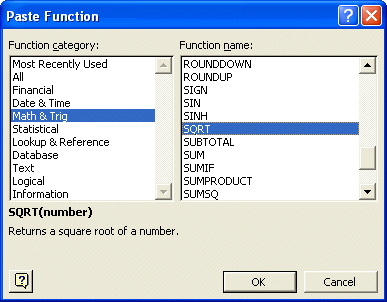You then need to select Math & Trig in the list on the left side (see above), and then SQRT function in the list on the right side.

Once the function is selected and once you clicked on OK, Excel displays a new dialog box, in which you need to enter the parameters of the function, here D2 (use the mouse to select cell D2). The result is pre-computed and displayed in the dialog box. It is displayed in cell F2 once you click on OK.If you want to know which formula has been used in an Excel cell, or if you want to modify the formula, you only need to click in the formula bar of Excel (or press F2) as the formula is displayed in it: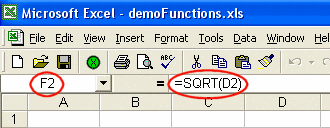## Using the XLSTAT_Var function

The following example involves two series of data: the first is the series of integers from 1 to 9, and the second corresponds to the first augmented by 1e9 (one billion).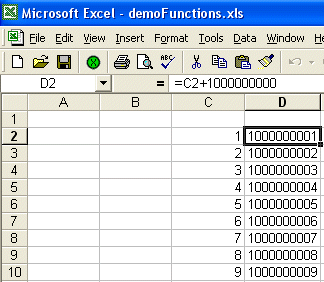The mean is 5 for the first series (5 + 1e9 = 1000000005) for the second series. But the variance should be the same for the two series as it measures the average deviation from the mean and as it is not sensitive to a translation of all the data.

We first decide to compute the unbiased variance (the sum of the squared deviations from the mean divided by n-1 where n is the number of observations), using the VAR function of Excel. The result displayed in cell C13 for the first series is correct (7.5), but it is wrong in cell D13 for the second series (except with Excel 2003).

We then compute the variance for the two series using the XLSTAT function.

To use an XLSTAT function, you only need to type = followed by its name or you can use the Insert / Function menu of Excel, and then choose XLSTAT in the list on the left. Then select the XLSTAT function in the list on the right.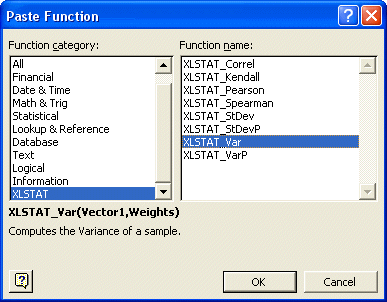We are using here the XLSTAT_Var function. The parameters which are displayed in bold are compulsory, while the other ones are optional. We select with the mouse the cells C2 to C10 for the first series, and the cells D2 to D10 for the second series.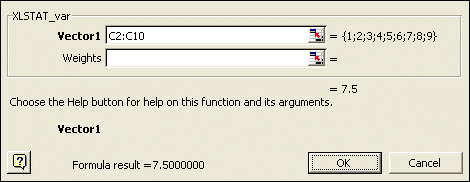We obtain the same result for both series.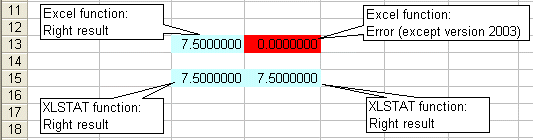As a conclusion, it is possible to use within Excel sheets functions that are based on the XLSTAT algorithms. We remind you that XLSTAT uses Excel only for inputting data and displaying results. All computations are done in independent programs and are based on proven algorithms.

#### Contact our technical support team: support@xlstat.com

https://cdn.desk.com/
false
desk